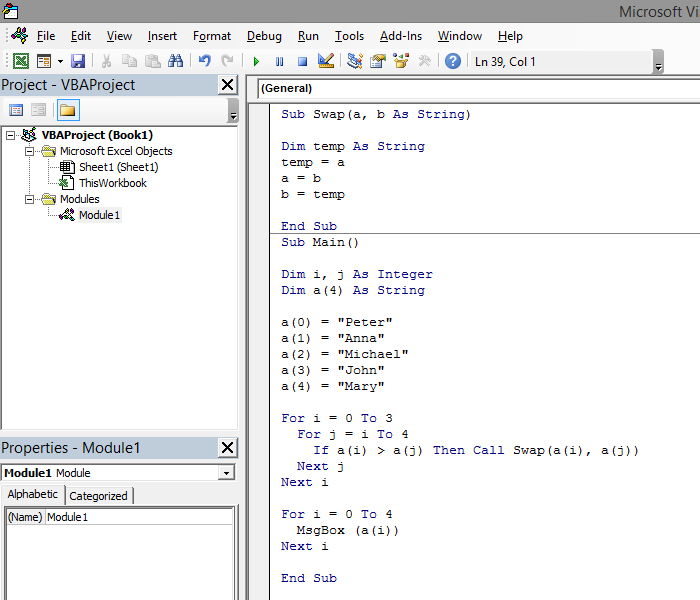# Sorting of an array

Sorting of an array is arranging of its members in a ascending or descending order. This can be achieved in many ways, and in the text that follows you will see one of the variants of the algorithm called Bubble Sort. It works by running two loops and then, depending on their index values, corresponding members of the array are compared. If the condition is met they change places. The procedure is repeated until the comparison is completed, and array sorted.

We created the Swap procedure, which changes places of two given arguments, and then used it in the Bubble Sort algorithm:

Sub Swap (a, b As String)

Dim temp As String
temp = a
a = b
b = temp

End Sub

Sub Main ()

Dim i, j As Integer
Dim a(4) As String

a(0) = “Petar”
a(1) = “Ana”
a(2) = “Mihailo”
a(3) = “Jovan”
a(4) = “Marija”

For i = 0 To 3
For j = i To 4
If a(i) > a(j) Then Call Swap(a(i), a(j))
Next j
Next i

For i = 0 To 4
MsgBox (a(i))
Next i

End Sub

If the operator in the condition is “>”, the sorting is done in ascending, and if it’s “<” in desecending order. After we start this program, the members of the array will be arranged in a ascending order.